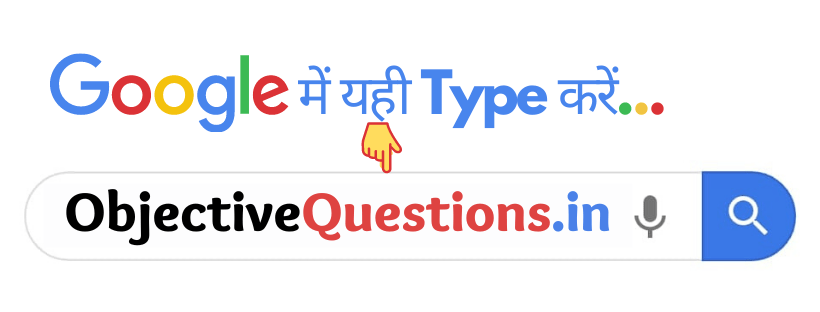# CHAPTER-13: KINETIC THEORY

1. A real gas behaves like an ideal gas if its –

• both pressure and temperature are high
• both pressure and temperature are low
• the pressure is high and the temperature is low
• the pressure is low and the temperature is high
the pressure is low and the temperature is high

2. Perfect gas equation –

• PV = RT
• PV = R/T
• PV = T/R
• PV = RV
PV = RT

3. Charles law –

• V/T = const
• VT = const
• PV = const
• V = 1/T
PV = const

4. Boyle’s law const

• V/T = const
• PV = const
• VT = const
• P = 1/V
PV = const

5. Degree of freedom for Monoatomie gas is –

• 3
• 5
• 8
• 7
3

6. Degree of freedom for Diatomic gas is –

• 3
• 8
• 7
• 5
5

7. The degree of freedom of a Triatemic gas is –

• 3
• 5
• 6
• 8
6

8. If the pressure and the volume of a certain quantity of ideal gas are doubled, then its temperature-

• become doubled
• become one – fourth
• remains constant
• become four times
become four times

9. If the pressure and the volume of a certain quality of ideal gas are hail, then its temperature –

• doubled
• becomes one fourth
• remains constant
• become four-time
becomes one fourth

10. The volume of a gas is increasing adiabatically. Its temperature –

• will increases
• will decreases
• will remains constant
• can increases or decreases